Home > A2C > Chapter 8 > Lesson 8.1.5 > Problem8-79

8-79.
1. Calculate each of the following values. Express your answers exactly and as decimal approximations. Homework Help ✎

1.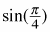2.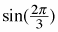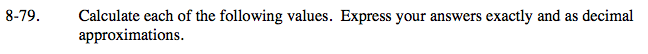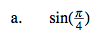$\text{Draw a unit circle and locate } \frac{\pi}{4} \text{ radians}.$

Remember that a full circle as 2π radians and a half circle has π radians.

$\text{How many degrees is equivalent to }\frac{\pi}{4} \text{ radians?}$

Draw a right triangle and use the special triangle relationship to find the exact value.

$\frac{\sqrt{2}}{2} \text{ or } 0.707$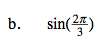$\text{Since } \pi \text{ is equivalent to } 180\degree, \frac{1}{3} \text{ of } \pi \text{ is } 60\degree.$

$\text{What is } \frac{2}{3} \text{ of } \pi? \text{ Locate } \frac{2\pi}{3} \text{ on the unit circle.}$

Draw a right triangle.
Notice that 60° is your reference angle.
Use the 30°-60°-90° relationship to find the exact value.